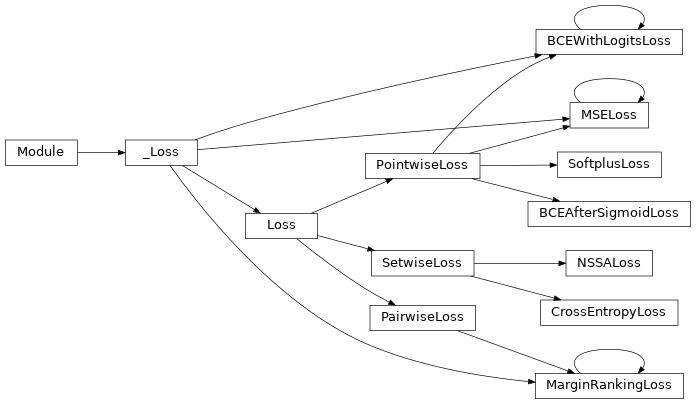# Loss Functions¶

Loss functions integrated in PyKEEN.

## Functions¶

 get_loss_cls(query) Get the loss class.

## Classes¶

 PointwiseLoss([size_average, reduce, reduction]) Pointwise loss functions compute an independent loss term for each triple-label pair. PairwiseLoss([size_average, reduce, reduction]) Pairwise loss functions compare the scores of a positive triple and a negative triple. SetwiseLoss([size_average, reduce, reduction]) Setwise loss functions compare the scores of several triples. BCEAfterSigmoidLoss([size_average, reduce, …]) A loss function which uses the numerically unstable version of explicit Sigmoid + BCE. BCEWithLogitsLoss([size_average, reduce, …]) A wrapper around the numeric stable version of the PyTorch binary cross entropy loss. CrossEntropyLoss([size_average, reduce, …]) Evaluate cross entropy after softmax output. MarginRankingLoss([margin, size_average, …]) A wrapper around the PyTorch margin ranking loss. MSELoss([size_average, reduce, reduction]) A wrapper around the PyTorch mean square error loss. NSSALoss([margin, adversarial_temperature, …]) An implementation of the self-adversarial negative sampling loss function proposed by [sun2019]. SoftplusLoss([reduction]) A loss function for the softplus.

## Class Inheritance Diagram¶